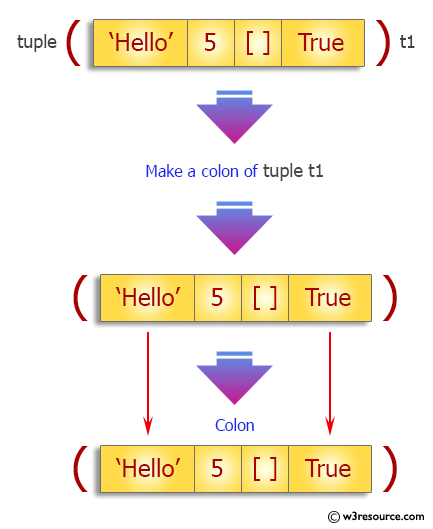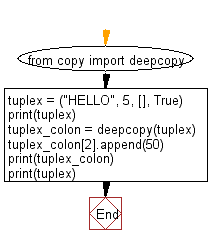﻿ Python: Colon of a tuple - w3resource# Python Exercise: Colon of a tuple

## Python tuple: Exercise-8 with Solution

Write a Python program to create the colon of a tuple.

Sample Solution:-

Python Code:

``````from copy import deepcopy
#create a tuple
tuplex = ("HELLO", 5, [], True)
print(tuplex)
#make a copy of a tuple using deepcopy() function
tuplex_colon = deepcopy(tuplex)
tuplex_colon.append(50)
print(tuplex_colon)
print(tuplex)
```
```

Sample Output:

```('HELLO', 5, [], True)
('HELLO', 5, , True)
('HELLO', 5, [], True)
```

Pictorial Presentation:Flowchart:## Visualize Python code execution:

The following tool visualize what the computer is doing step-by-step as it executes the said program:

Python Code Editor:

Have another way to solve this solution? Contribute your code (and comments) through Disqus.

What is the difficulty level of this exercise?

Test your Programming skills with w3resource's quiz.

﻿

## Python: Tips of the Day

Decapitalizes the first letter of a string:

Example:

```def tips_decapitalize(s, upper_rest=False):
return s[:1].lower() + (s[1:].upper() if upper_rest else s[1:])
print(tips_decapitalize('PythonTips'))
print(tips_decapitalize('PythonTips', True))
```

Output:

```pythonTips
pYTHONTIPS
```